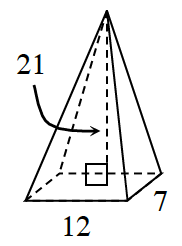### Home > CCG > Chapter 11 > Lesson 11.1.3 > Problem11-52

11-52.

Multiple Choice: Calculate the volume of the rectangle-based pyramid at right.1. $84\text{ units}^3$

1. $648\text{ units}^3$

1. $882\text{ units}^3$

1. $1764\text{ units}^3$

1. None of these

$\text{Volume}=\frac{1}{3}(\text{area of base})(\text{height})$